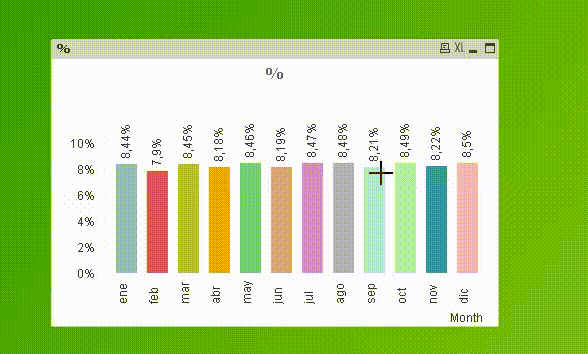New to QlikView

Discussion board where members can get started with QlikView.

Announcements
QlikWorld 2020: Join us May 11 - 14, 2020 in Phoenix, AZ. Register early and save \$400. Learn More
HighlightedNot applicable

Showing percentages in qlikview

Hi All

I'm using the below expression to calculate the percentage values

=sum({<my={'1'}>}sales)/sum({<my={'1'}>}total(sales)) *100

and my output values are

9.683284295

8.614611669

i want it to be rounded to two decimals and a %age symbol ate the end

9.68 %

8.61% i want like this

and for the below expression

=round(sum(sales)/sum(total(sales)100,0.01)

also i want the values as same rounded to two decimals ans a %age symbol

7 Replies
Valued Contributor

Re: Showing percentages in qlikview

Hi,

:Honored Contributor

Re: Showing percentages in qlikview

Hi John,

Don't bother multiplying by 100. take that out so you have a decimal fraction then format the result by wrapping it in the num function like this:

=num(YourExpressionHere,'0.00%')

cheers

AndewMVP

Re: Showing percentages in qlikview

=Num(Round(sum({<my={'1'}>}sales)/sum({<my={'1'}>}total sales),0.0001),'0.00%')

or to only format with two decimal places (not changing the actual value):

=Num(sum({<my={'1'}>}sales)/sum({<my={'1'}>}total sales),'0.00%')

hope this helps

regards

MarcoNot applicable

Re: Showing percentages in qlikview

Hi Macro

Can you explain me how it is working

the logic behind the

,0.0001),'0.00%')

and

if you have any idea on this can u please help me out

One of the toughest requirement is, comparing the top n values in current period to the same top n values in the previous period and pre previous period can you please help us in this

The easiest way to achive and the logical way.MVP

Re: Showing percentages in qlikview

Hi,

Round( xxx , 0.0001)   rounds to the nearest multiple of 0.01%, while Num( xxx , '0.00%') formats a number as percentage with 2 decimal places.

hope this helps

regards

Marco

Valued Contributor III

Re: Showing percentages in qlikview

Hi John,

Here is the way,

Right Click on your Chart > Chart Properties > Select your expression > Select Checkbox "Relative" > Apply > OK.

Kind regards,

Ishfaque Ahmed

New Contributor

Re: Showing percentages in qlikview

I was struggling to get the correct percentage but your comment suggesting "not to multiply by 100" solved it. Thanks!